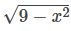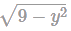The range of the real-valued function f(x) =...

### Related TestThe range of the real-valued function f(x) =is:Kunaal SatijaGiven: f(x) =Let, y2 = 9 - x2
⇒ x2 = 9 - y2
x =...(1)
We know that for any function f(x)=,y ≥ 0…. (A)
From equation (1).
9 − y2 ≥ 0
y2 − 9 ≤ 0
⇒ (y + 3)(y − 3) ≤ 0
⇒ −3 ≤ y ≤ 3
But from equation (A) y must be positive, then, y = [0, 3].
Range = the value of y = [0, 3]
Hence, the correct answer is [0, 3].View courses related to this question Explore CAT courses
 Explore CAT coursesView courses related to this question1 Crore+ students have signed up on EduRev. Have you?

• ### ‘f’ is a real function such that f(x + y) = f(xy) for all real ... more(Scan QR code)